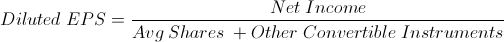# Diluted Earnings per Share

Diluted EPS Calculator (Click Here or Scroll Down)Diluted earnings per share, or Diluted EPS, is a firm's net income divided by the sum of it's average shares and other convertible instruments.

A company's net income can be found on its income statement.

A company's average shares refers to the weighted average of common shares throughout the year. The weights of each factor would be the length of time each quantity of common shares is outstanding.

As a simple example, suppose that a company has 100 outstanding common shares for 9 months and 120 outstanding common shares for 3 months. The weights of 9 months and 3 months would be .75 and .25 respectively. This represents 3/4's of a year and 1/4 of a year. Thus, the formula for the average shares portion of the diluted earnings per share for this example would be .75(100) + .25(120), which would equal a weighted average of 105 common shares for the entire year.

## Other Convertible Instruments and Diluted EPS

The term "convertible instruments" refers to any financial instrument that could possibly be converted into a common shares

For reference, a few examples of convertible instruments that may be considered in the diluted earnings per share formula are stock options and convertible preferred stocks, but there are many others and anything than has the availability to be converted to a common share could be included.

## Use of Diluted EPS

The diluted earnings per share is used by investors in replace of earnings per share to account for financial instruments that can be converted to shares. This conservative approach to calculating earnings per share may be used in lieu of the simple EPS formula as any convertible instrument could be converted at any time which could cause the real events to widely deviate from the simple EPS future estimates. This is especially important to consider when other financial formulas use earnings per share in its calculations.

New to Finance?